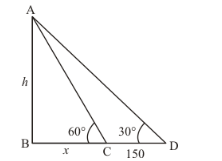# The angle of elevation of a tower from a point on the same level as the foot of the tower is 30°

Question:

The angle of elevation of a tower from a point on the same level as the foot of the tower is $30^{\circ}$. On advancing 150 metres towards the foot of the tower, the angle of elevation of the tower becomes $60^{\circ}$. Show that the height of the tower is $129.9$ metres (Use $\sqrt{3}=1.732$ )

Solution:

Let h be height of tower and angle of elevation of foot of tower is 30°, on advancing
150 m towards the foot of tower then angle of elevation becomes 60°.

We assume that BC = x and CD = 150 m.

Now we have to prove height of tower is 129.9 m.

So we use trigonometrical ratios.In a triangle,

$\Rightarrow \quad \tan C=\frac{A B}{B C}$

$\Rightarrow \quad \tan 60^{\circ}=\frac{A B}{B C}$

$\Rightarrow \quad \sqrt{3}=\frac{h}{x}$

$\Rightarrow \quad \frac{h}{\sqrt{3}}=x$

Again in a triangle,

$\Rightarrow \quad \tan D=\frac{A B}{B C+C D}$

$\Rightarrow \quad \tan 30^{\circ}=\frac{h}{x+150}$

$\Rightarrow \quad \frac{1}{\sqrt{3}}=\frac{h}{x+150}$

$\Rightarrow \quad x+150=\sqrt{3} h$

$\Rightarrow \quad x=\sqrt{3} h-150$

$\Rightarrow \quad \frac{h}{\sqrt{3}}=\sqrt{3} h-150$

$\Rightarrow \quad h=3 h-150 \sqrt{3}$

$\Rightarrow \quad 2 h=150 \sqrt{3}$

$\Rightarrow \quad h=\frac{150 \times 1.732}{2}$

$\Rightarrow \quad h=129.9$

Hence the height of tower is $129.9 \mathrm{~m}$ proved.